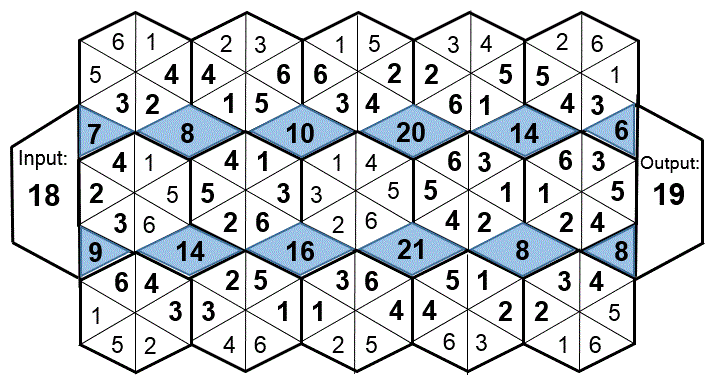Select Page

Each month, a new set of puzzles will be posted.  Come back next month for the solutions and a new set of puzzles, or subscribe to have them sent directly to you.

## MIND-Xpander Logic Problems:

1. Ship Ahoy – A ship is at anchor. Over its side hangs a rope ladder with rungs a foot apart. The tide rises at the rate of 8 inches per hour. If the ladder was 8 feet above the water level when the tide began to rise, how much of the rope ladder will remain above water after 6 hours?
2. Water lilies on a certain lake double in area every twenty-four hours. From the time that the first water lily appears until the lake is completely covered takes 60 days. On what day is the lake half-covered?

## EQUATE-Times Puzzles

The missing numbers are between 1 & 9 with no repeats. The three numbers in each row, when multiplied or times together, equals the totals on the right, and the columns, when multiplied together, equals the totals along the bottom. The multiples of the two diagonals are also given. Solve the puzzle by finding all of the missing numbers that satisfy all of the multiplied results as shown?

A.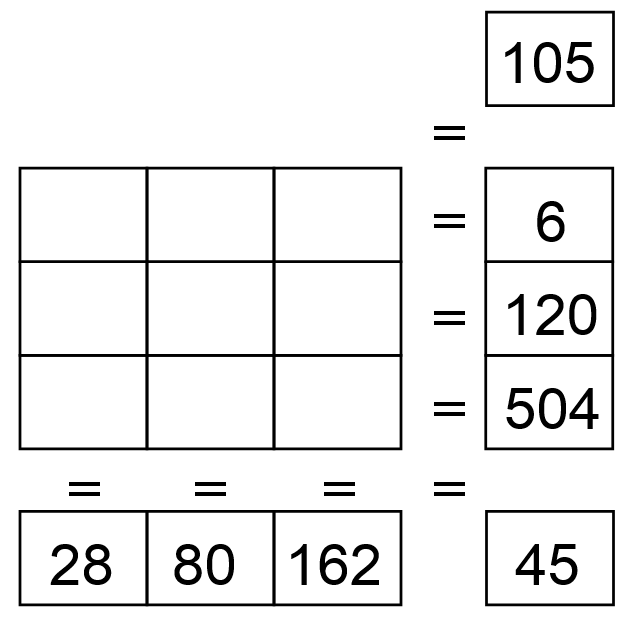B.## HEXAGON-numeric Puzzle (Level 3)

Fit the numbers 1 – 6 in each hexagon and where the hexagon sides touch each other, the numbers in these 2 segments will be the same. No number can be repeated in a hexagon. The numbers in the shaded areas are the sum of the numbers in the 2 or 4 segments that they side with. The numbers in the ‘Input’ and ‘Output’ boxes are the sum of the numbers in the 3 triangular segments that they side with. The numbers in the ‘Sum’ boxes are the sum of the numbers in the 4 segments that they side with. Solve the puzzle and find the ‘Output’ number.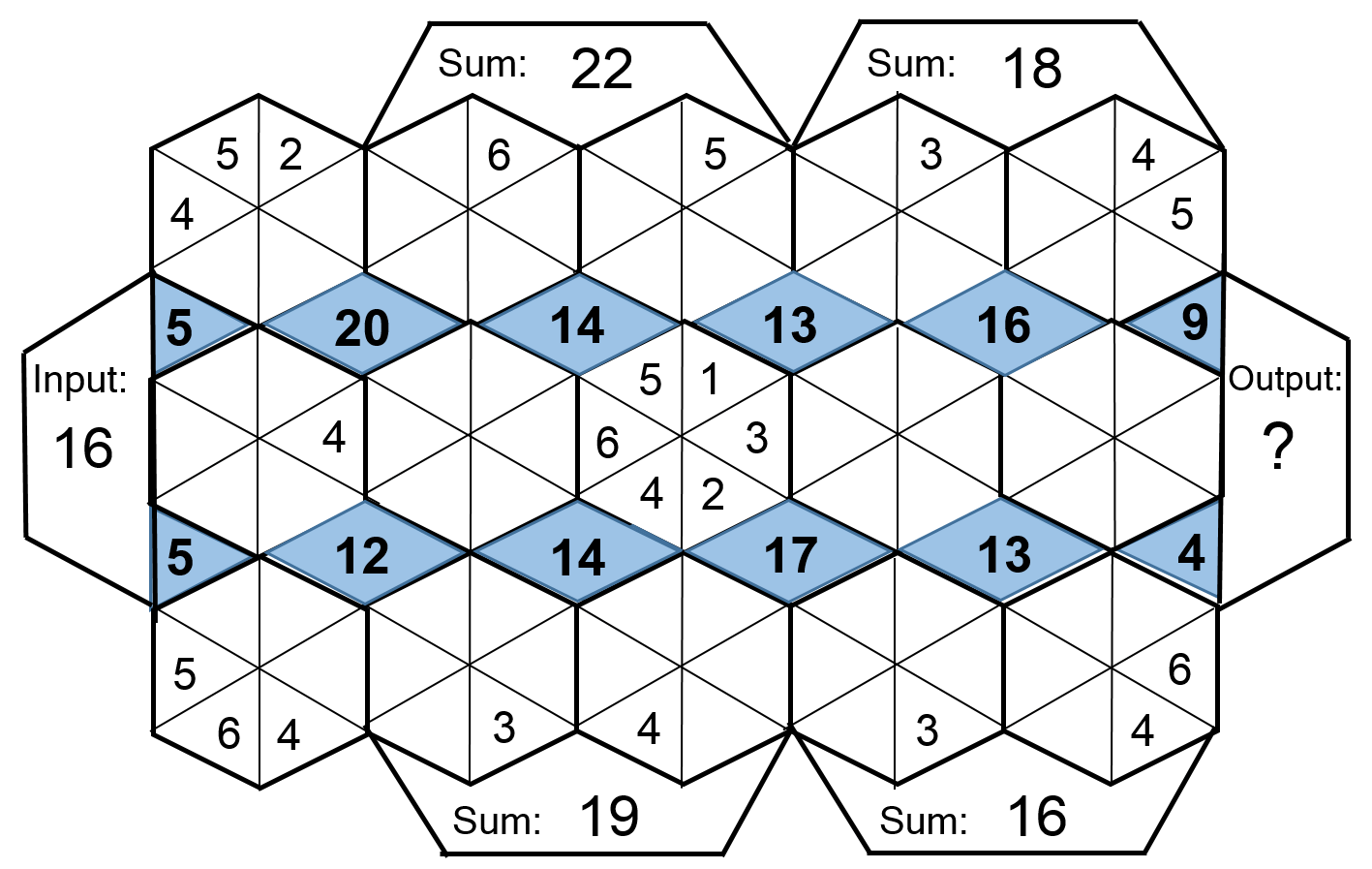## Feedback

There are more than one way of doing these puzzles and may well be more than one answer.  Please let me and others know what alternatives you find by commenting below.  We also welcome general comments on the subject and any feedback you'd like to give.

If you have a question that needs a response from me or you would like to contact me privately, please use the contact form.

## Get more puzzles!

If you've enjoyed doing the puzzles, consider ordering the book; 150+ of the best puzzles in a handy pocket sized format. Click here for full details.

## MIND-Xpander Logic Problem:

There are five people in a room and each shakes every other person’s hand exactly one time. How many total handshakes will there be?

Solution = 10

You can draw a pentagon-star diagram where each number represents a person and the lines/handshapes show their interactions. Just add up the lines (10)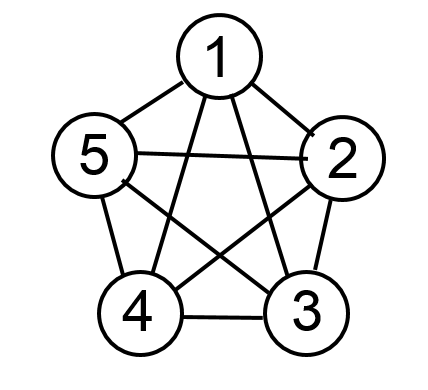Or, use this handy formula to determine the total number of handshakes (where n = number of people): [n x (n-1)] / 2 = ? [5 x 4] / 2 = 10

## ROMAN Arithmetic Puzzles

Solve the following arithmetic problems and state your answers in Roman Numerals. Letters representing numbers in the Roman numerical system: I = 1, V = 5, X = 10, L = 50, C = 100, D = 500, M = 1,000. In this system, a letter placed after another of greater value adds (thus XVI is 16) whereas a letter placed before another of greater value subtracts (thus XC is 90).

Solutions: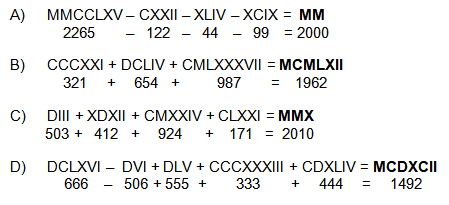## HEXAGON-numeric Puzzle (Level 2)

Fit the numbers 1 – 6 in each hexagon and where the hexagon segments touch each other, their numbers in these 2 segments will be the same. No number can be repeated in a hexagon. The numbers in the shaded areas are the sum of the numbers in the 2 or 4 segments that they side with. The numbers in the ‘Input’ and ‘Output’ boxes are the sum of the numbers in the 3 triangular segments that they side with. Solve the puzzle and find the ‘Output’ number (?).

Solution: